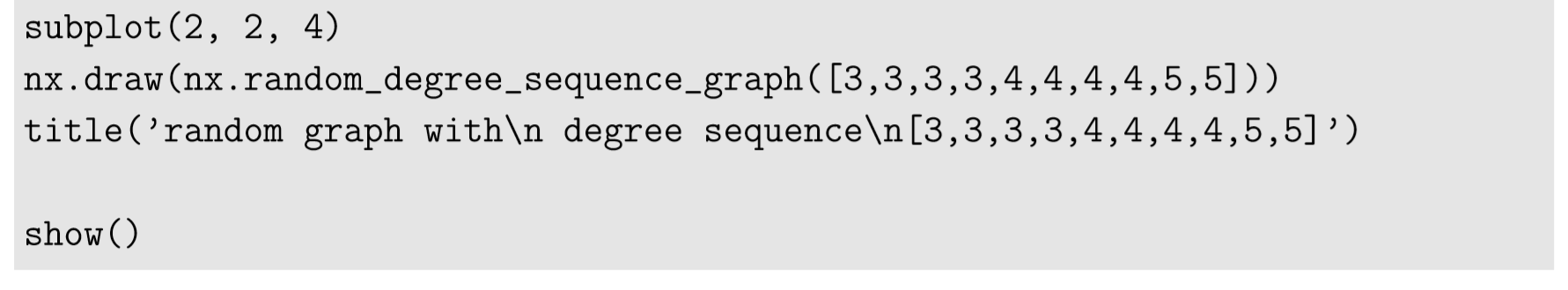$$\newcommand{\id}{\mathrm{id}}$$ $$\newcommand{\Span}{\mathrm{span}}$$ $$\newcommand{\kernel}{\mathrm{null}\,}$$ $$\newcommand{\range}{\mathrm{range}\,}$$ $$\newcommand{\RealPart}{\mathrm{Re}}$$ $$\newcommand{\ImaginaryPart}{\mathrm{Im}}$$ $$\newcommand{\Argument}{\mathrm{Arg}}$$ $$\newcommand{\norm}{\| #1 \|}$$ $$\newcommand{\inner}{\langle #1, #2 \rangle}$$ $$\newcommand{\Span}{\mathrm{span}}$$

15.6: Generating Random Graphs

$$\newcommand{\vecs}{\overset { \rightharpoonup} {\mathbf{#1}} }$$

$$\newcommand{\vecd}{\overset{-\!-\!\rightharpoonup}{\vphantom{a}\smash {#1}}}$$

So far, we have been creating networks using deterministic methods, e.g., manually adding nodes and edges, importing data ﬁles, etc. In the meantime, there are some occasions where you want to have a randomly generated network. Here are some examples of NetworkX’s built-in functions that can generate random graph samples:The output is shown in Fig. 15.10. The ﬁrst example, gnm_random_graph(n, m), simply generates a random graph made of n nodes and m edges. The second example, gnp_random_graph(n, p), generates a random graph made of n nodes, where each node pair is connected to each other with probability p. These two types of random graphs are called Erd˝os-R´enyi random graphs after two Hungarian mathematicians Paul Erd˝os and Alfr´ed R´enyi who studied random graphs in the 1950s . The third example, random_regular_graph(k, n), generates a random regular graph made of n nodes that all have the same degree k. The fourth example, random_degree_sequence_graph(list), generates a random graph whose nodes have degrees speciﬁed in list. In this particular example, four nodes have degree 3, four nodes have degree 4, and two nodes have degree 5. Note that this function may not be able to generate a graph within a given number of trials (which is set to 10 by default). You can increase the number of trials by specifying the tries option in the function, e.g., tries = 100.Figure $$\PageIndex{1}$$: Visual output of Code 15.21.

Random graphs can also be generated by randomizing existing graph topologies. Such network randomization is particularly useful when you want to compare a certain property of an observed network with that of a randomized “null” model. For example, imagine you found that information spreading was very slow in the network you were studying. Then you could test whether or not the network’s speciﬁc topology caused this slowness by simulating information spreading on both your network and on its randomized counterparts, and then comparing the speed of information spreading between them. There are several different ways to randomize network topologies. They differ regarding which network properties are to be conserved during randomization. Some examples are shown below (here we assume that g is the original network to be randomized):

gnm_random_graph(g.number_of_nodes(), g.number_of_edges()) conserves the numbers of nodes and edges, nothing else.
random_degree_sequence_graph(g.degree().values()) conserves the sequence of node degrees, in addition to the numbers of nodes and edges. As noted above, this function may not generate a graph within a given number of trials.
expected_degree_graph(g.degree().values()) conserves the number of nodes and the sequence of “expected” node degrees. This function can always generate a graph very quickly using the efﬁcient Miller-Hagberg algorithm. While the generated network’s degree sequence doesn’t exactly match that of the input (especially if the network is dense), this method is often a practical, reasonable approach for the randomization of large real-world networks.
double_edge_swap(g) conserves the numbers of nodes and edges and the sequence of node degrees. Unlike the previous three functions, this is not a graph-generating function, but it directly modiﬁes the topology of graph g. It conducts what is called a double edge swap, i.e., randomly selecting two edges a − b and c − d, removing those edges, and then creating new edges a−c and b−d (if either pair is already connected, another swap will be attempted). This operation realizes a slight randomization of the topology of g, and by repeating it many times, you can gradually randomize the topology from the original g to a completely randomized network. double_edge_swap(g, k) conducts k double edge swaps. There is also connected_double_edge_swap available, which guarantees that the resulting graph remains connected.

Exercise $$\PageIndex{1}$$

RandomizethetopologyofZachary’sKarateClubgraphbyusing each of the following functions and visualize the results:

gnm_random_graph

random_degree_sequence_graph

expected_degree_graph

double_edge_swap (5 times)

double_edge_swap (50 times)